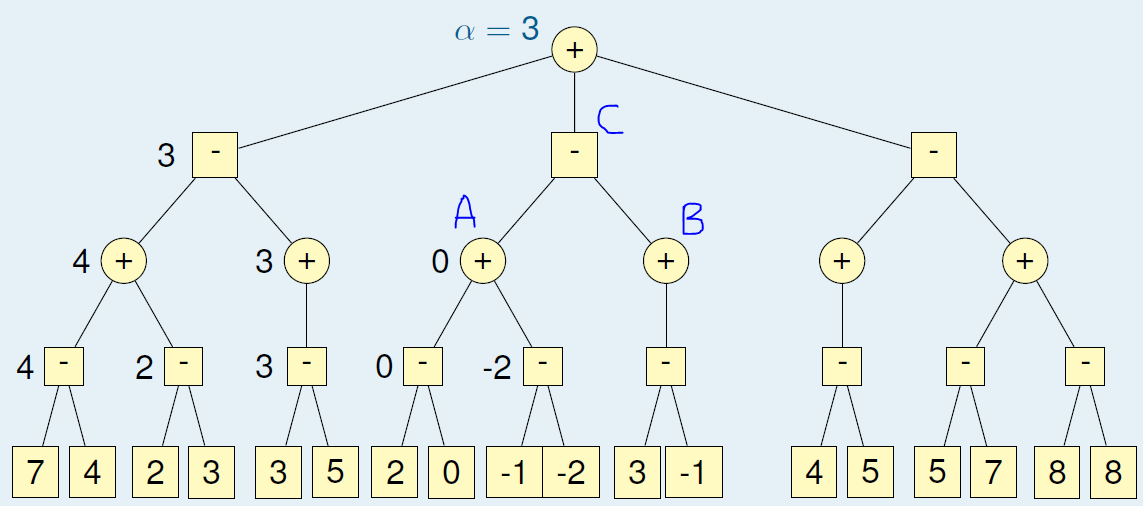# Make your own AI Game Engine – 3

So, I hope you have read the two previous articles, part 1 and part 2 of this. I promised to explain about Alpha-Beta pruning, and here we go.

# Alpha-Beta Pruning

In normal mini-max algorithm, we have to traverse through all the nodes and evaluate them. But sometime we don’t have to, if we already got a better option than the one we’re going to examine.

Let’s and example game tree.Sample Game Tree

When we finish the 1st sub-tree, we will get the max value, we call it alpha (α), as 3.Game Tree : 1st Sub-Tree Traversed

In here alpha is the maximum value for a node, and beta is minimum value for a node.

Then we continue to traverse through the 2nd sub-tree. And at some point, the values will be like following.Game Tree : Traversing 2nd Sub-Tree

When we come to this point, that we know the maximum value we will get to the root of the 2nd sub-tree is 0. The reason is, if there are values above 0 in the branch B, it will be ignored because C takes the minimum value. If there are lower values than 0 in the branch B, C will take them, meaning the maximum value that C will take is 0. But we already know that the parent of C is a max node and it has alpha = 3. So there is no possibility that it will select a lower value than 3. Hence, at this point, we can stop traversing the sub-tree of C by simply returning 0 to the root.

The same scenario is applied when there is a max node at C, but the other way-round.

To reduce the complexity, I will use two functions for Min and Max nodes. However the Alpha-Beta search will be look like:

```function ALPHABETA(search tree node s)
return MAX_VALUE(s,−∞,+∞)
end function```

We call the max function at the root, since we need the max value at the root node. But if we’re taking this as 3 sub-trees (as I explained here), we have to apply MIN_VALUE for each of the children of the root, and get the child with the max value.

Here are the pseudo code for the MIN_VALUE and MAX_VALUE functions.

```function MIN_VALUE(node s, α, β))
if s is a leaf then
return utility(s)
end if
value = +∞ // initialize β as the highest possible value
for each child t of s :
value = min(value, MAX_VALUE(t, α, β)) // go to next level of the tree
if value ≤ α then
return value
end if
β = min(β, value)
end for
return value
end function```
```function MAX_VALUE(node s, α, β))
if s is a leaf then
return utility(s)
end if
value = −∞ // initialize α as the lowest possible value at the beginning
for each child t of s :
value = max(value, MIN_VALUE(t, α, β)) // go to next level of the tree
if value ≥ β then
return value
end if
α = max(α, value)
end for
return value
end function```

No matter how much this performs, if we still create the whole tree before we traverse, it will cost us a LOT. So it’s better to create the children on the way of traversal.

# Quick Comparison

• Mini-Max visits all tree nodes. With branching factor b and depth d, this amounts to bd.
• Number of nodes that Alpha-Beta visits, depends on the order in which moves are examined.
• If the best move is always examined first, Alpha-Beta visits bd/2 nodes.
• Hence, we can use domain-specific knowledge to order the possible moves to achieve more efficiency.

For the details about the utility function, please see the edit of my first article.